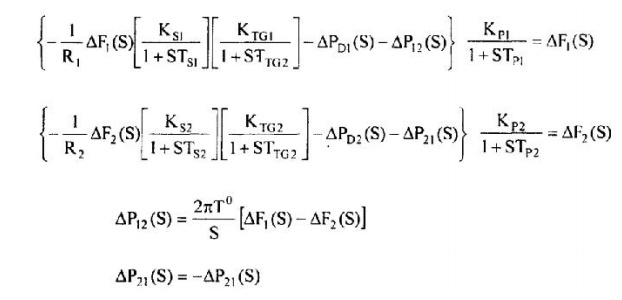# Dynamic Response

Let us now turn our attention during the transient period for the sake of simplicity. We shall assume the two areas to be identical.

DYNAMIC RESPONSE

Let us now turn our attention during the transient period for the sake of simplicity. We shall assume the two areas to be identical. Further we shall be neglecting the time constants of generators and turbines as they are negligible as compared to the time constants of power systems. The equation may be derived for both controlled and uncontrolled cases. There are four equations with four variables, to be determined for given PDl and PD2. The dynamic response can be obtained; even though it is a little bit involved. For simplicity assume that the two areas are equal. Neglect the governor and turbine dynamics, which means that the dynamics of the system under study is much slower than the fast acting turbine-governor system in a relative sense. Also assume that the load does not change with frequency (D, = D2 = D = 0).We obtain under these assumptions the following relationsØ Note t hat both K and ro2 are positive. From the roots of the characteristic equation we noticeth the system is stable and damped.

Ø The frequency of the damped oscillations is given by Since Hand fo are constant, the frequency of oscillations depends upon the regulation parameter R. Low R gives high K and high damping and vice versa .

Ø   We thus conclude from the preceding analysis that the two area system, just as in the case of a single area system in the uncontrolled mode, has a steady state error but to a lesser extent and the tie line power deviation and frequency deviation exhibit oscillations that are damped out later.

Study Material, Lecturing Notes, Assignment, Reference, Wiki description explanation, brief detail
Power System Operation and Control : Real Power Frequency Control : Dynamic Response |